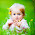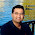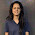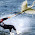### What is Discrete Convolution?

Let's assume a system which is linear time invariant (LTI) in nature.
If y1(t) is the output of the system corresponding to the input x1(t) and y2(t) is the output of the system corresponding to the input x2(t) in the continuous domain.

x1(t)  <>  y1(t)
x2(t)  <>  y2(t)
Then the system will be LTI if
ax1(t) + bx2(t)  <>  ay1(t) + by2(t)
Now let's think about the impulse response of a system. Impulse response is the output of the system when input is a simple impulse at time zero.

Now let's discuss about the discrete time function.
Let's assume our system has an impulse response [4,2,3,0,0,0,0].

This means at time t = 0 the impulse response is 4, at t = 1 the impulse response is 2, at t = 2 the impulse response is 3 and after that it is zero till the infinity.
As the system is causal because there is no output before the input is given. i.e. the output of the system started at t=0.
In short, for an input [1,0,0,0,0,0] (impulse input at t = 0) the output of the system is [4,3,2,0,0,0,0,0].

Now instead of impulse at t=0 let's provide the impulse at t=1, that means now the input is [0,1,0,0,0,0]. In short you have shifted the input by t=1.
As the system is time invariant so the output will also be shifted by t=1. So, the output of the system will be [0,4,3,2,0,0,0,0,0]. Now the output of the system at t=0 is 0 because the system is causal and no input is given at t=0.

Now multiply the input by 2. that means now the input is [0,2,0,0,0,0].
As the system is linear, so the output will also be multiplied by the same factor 2.

Now the output will be [0,8,6,4,0,0,0,0].

Now apply the superposition theorem and add both the inputs.
[1,0,0,0,0] + [0,2,0,0,0] = [1,2,0,0,0]

As the system is linear so output will be the sum of both the outputs.
[4,3,2,0,0,0] + [0,8,6,4,0,0] = [4,11,8,4,0,0,0]

As a result for the input [1,2,0,0] the output of the system is [4,11,8,4,0,0].

Now we have:
x[n] = [1,2]
h[n] = [4,3,2]
So, as the definition of the convolution the output of the system will be:
y[n] = x[n]*h[n]
y[n] = [1,2]*[4,3,2]

Here y[n] will not be a simple multiplication of x[n] and h[n].
How will you multiply two sequences?

So as a result we have:
y[n] = [y, y, y, y]
y[n] = [4,11,8,4]

That means convolution is nothing but just the multiplication of two sequences or polynomial

To explain this let us assume two polynomials p and q.

p = ax + b;
q = cx^2 + dx + e;

Now let's just multiply them:
p*q = (ax + b)*(cx^2 + dx + e);
p*q = acx^3 + x^2(ad+bc) + x(bd + ae) + be;
Now replace both polynomial coefficients with our previous x[n] and h[n].
Coefficients of p =  (a,b)   = x[n] = [1,2]
Coefficients of q = (c,d,e) = h[n] = [4,3,2]

y[n] = coefficients of p*q = [ac, (ad+bc), (bd+ae), be];
= [4, (3+8), (6+2), 4];
= [4,11,8,4]

And ye :) it is same as above.

That means Convolution is just a Polynomial Multiplication

1.I have never read such a good explanation :) . Thank you very much. Waiting for DFT and FFT.

2.Thanks a lot Mr. Sakshama. Finally I understood. =))

3.4.Really nice interpretation (Y)

5.phod dala bhai.......